Home
Hostname: page-component-79b67bcb76-ncjtf Total loading time: 0.202 Render date: 2021-05-16T07:15:11.613Z Has data issue: true Feature Flags: { "shouldUseShareProductTool": true, "shouldUseHypothesis": true, "isUnsiloEnabled": true, "metricsAbstractViews": false, "figures": false, "newCiteModal": false, "newCitedByModal": true, "newEcommerce": true }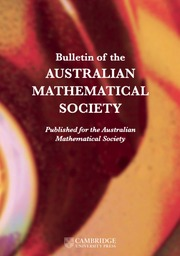Bulletin of the Australian Mathematical Society

## Abstract

We extend known results concerning crossing numbers by giving the crossing number of the join product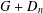$G+D_{n}$ , where the connected graph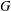$G$ consists of one$4$ -cycle and of two leaves incident with the same vertex of the$4$ -cycle, and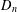$D_{n}$ consists of$n$ isolated vertices. The proofs are done with the help of software that generates all cyclic permutations for a given number$k$ and creates a graph for calculating the distances between all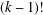$(k-1)!$ vertices of the graph.

## MSC classification

Type
Research Article
Information
Bulletin of the Australian Mathematical Society , December 2018 , pp. 353 - 362
© 2018 Australian Mathematical Publishing Association Inc.

## Access options

Get access to the full version of this content by using one of the access options below.

## Footnotes

The research was supported by the internal faculty research project no. FEI-2017-39.

## References

Berežný, Š., Buša, J. Jr and Staš, M., ‘Software solution of the algorithm of the cyclic-order graph’, Acta Electrotech. Inform. 18(1) (2018), 310.CrossRefGoogle Scholar
Berežný, Š. and Staš, M., ‘On the crossing number of the join of five vertex graph G with the discrete graph D n ’, Acta Electrotech. Inform. 17(3) (2017), 2732.CrossRefGoogle Scholar
Berežný, Š. and Staš, M., ‘Cyclic permutations and crossing numbers of join products of symmetric graph of order six’, Carpathian J. Math. 34(2) (2018), 143155.Google Scholar
Hernández-Vélez, C., Medina, C. and Salazar, G., ‘The optimal drawing of K 5, n ’, Electron. J. Combin. 21(4) (2014), P4.1, 29 pages.Google Scholar
Kleitman, D. J., ‘The crossing number of K 5, n ’, J. Combin. Theory 9 (1970), 315323.CrossRefGoogle Scholar
Klešč, M., ‘The join of graphs and crossing numbers’, Electron. Notes Discrete Math. 28 (2007), 349355.CrossRefGoogle Scholar
Klešč, M., ‘The crossing number of join of the special graph on six vertices with path and cycle’, Discrete Math. 310 (2010), 14751481.CrossRefGoogle Scholar
Klešč, M., Petrillová, J. and Valo, M., ‘On the crossing numbers of Cartesian products of wheels and trees’, Discuss. Math. Graph Theory 71 (2017), 339413.Google Scholar
Klešč, M. and Schrötter, Š., ‘The crossing numbers of join products of paths with graphs of order four’, Discuss. Math. Graph Theory 31 (2011), 312331.CrossRefGoogle Scholar
Klešč, M. and Schrötter, Š., ‘The crossing numbers of join of paths and cycles with two graphs of order five’, in: Mathematical Modeling and Computational Science, Lecture Notes in Computer Science, 7125 (Springer, Berlin–Heidelberg, 2012), 160167.CrossRefGoogle Scholar
Staš, M., ‘On the crossing number of the join of the discrete graph with one graph of order five’, Math. Model. Geom. 5(2) (2017), 1219.CrossRefGoogle Scholar
Staš, M., ‘Cyclic permutations: crossing numbers of the join products of graphs’, in: Proc. APLIMAT 2018: 17th Conf. Applied Mathematics (Slovak University of Technology, Bratislava, 2018), 979987.Google Scholar
Woodall, D. R., ‘Cyclic-order graphs and Zarankiewicz’s crossing number conjecture’, J. Graph Theory 17 (1993), 657671.CrossRefGoogle Scholar

# Send article to Kindle

Note you can select to send to either the @free.kindle.com or @kindle.com variations. ‘@free.kindle.com’ emails are free but can only be sent to your device when it is connected to wi-fi. ‘@kindle.com’ emails can be delivered even when you are not connected to wi-fi, but note that service fees apply.

Find out more about the Kindle Personal Document Service.

DETERMINING CROSSING NUMBERS OF GRAPHS OF ORDER SIX USING CYCLIC PERMUTATIONS
Available formats
×

# Send article to Dropbox

To send this article to your Dropbox account, please select one or more formats and confirm that you agree to abide by our usage policies. If this is the first time you use this feature, you will be asked to authorise Cambridge Core to connect with your <service> account. Find out more about sending content to Dropbox.

DETERMINING CROSSING NUMBERS OF GRAPHS OF ORDER SIX USING CYCLIC PERMUTATIONS
Available formats
×

# Send article to Google Drive

To send this article to your Google Drive account, please select one or more formats and confirm that you agree to abide by our usage policies. If this is the first time you use this feature, you will be asked to authorise Cambridge Core to connect with your <service> account. Find out more about sending content to Google Drive.

DETERMINING CROSSING NUMBERS OF GRAPHS OF ORDER SIX USING CYCLIC PERMUTATIONS
Available formats
×
×